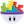#### Howdy, Stranger!

It looks like you're new here. If you want to get involved, click one of these buttons!

# decrease moves based on score

hello everyone
so i bought the squares template and im working around with it
so i figured ill make levels and have different number of moves in each level

so basically i figured the only way to detect number of moves is based on the score
but the score doesnt only change by +1 its +1 on for each square that is matched so if its 4 its +4 if its 5 its +5 and so on

so when i put it in a rule the moves was decreased exactly by the number of score which is what i did basically
how do i make it so that when the score changes but not by specific number just the fact the it changed meaning the player did make a successful move, the number of moves decreases?

i hope you understood

• Posts: 573Member, BASICedited October 2017

Hi Rawan,

You are welcome to continue to the email message you started in August. (I imagine its the same project)

There are a few ways in which you can add in moves. The following is the most simple.

You will need to create a new actor.
In this actor, you will need to the following rule:

When touch.count = 0
(And)
When game.Count >= 2

Then change Moves (Attribute) to minus 1.

Then create a new seperate rule.

When Touch.Count = 0

Add a timer in, set to "After" a value of 0.06
Inside the behaviour, you will need a change attribute behaviour and change the game.Count attribute to a value of zero.

What is happening, is that the squares will not be removed until there is no touches on the screen. (Touch count = 0)

And the squares will only disappear/fall when there is 2 or more squares selected.
(Game.Count >= 2)

Hope that helps.
Best,
Two.E

• didnt worki think i did something wrong

• Posts: 573Member, BASICHello,

Perhaps that is the issue.
If it doesn't work then, I will send you over my file.

Best,

• @Two.E said:
Hello,